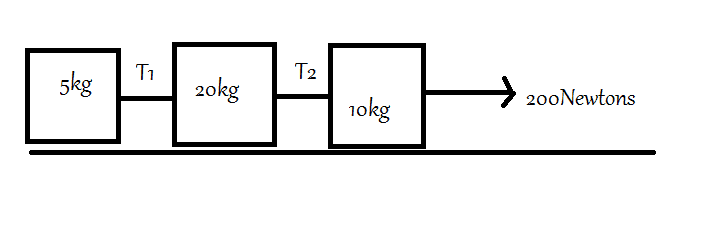# What is the tension in string?

## Homework Statement

We have to find T1 and T2From right to left objects are: A B C

F=ma

## The Attempt at a Solution

Okay. So I found acceleration of whole system:
F=ma
200N= (20+10+5)*a
200/35=a
a= 40/7 ms-2

Now, finding T1 is fairly simple:
f=ma
T1=5*(40/7)
T1=200/7 Newtons (Let's not reduce it right now to decimals)

Now, T2. This is where the problem arises.
T2 can be calculated by the difference of the forces on object A.
f=ma
Force on A= 10kg*(40/7)
= 400/7 Newtons

T2 will be:
200-T2= 400/7
200-400/7= T2
1000/7 Newtons= T2

1000/7N= 142.75 Newtons.

#### Attachments

Last edited:

Orodruin
Staff Emeritus
Homework Helper
Gold Member
2021 Award
It is not clear from your description what A is, bit it seems to be the 10 kg mass to the right by your attempt.

200= T2- 400/7 (T2 is greater than 400/7 N but obviously lesser than 200 N)
200-400/7= T2
Apart from the fact that you made a sign error in the first that mysteriously disappeared in the second step, yes, this is a viable approach.

It is not clear from your description what A is, bit it seems to be the 10 kg mass to the right by your attempt.

Apart from the fact that you made a sign error in the first that mysteriously disappeared in the second step, yes, this is a viable approach.
Thanks a bunch for: